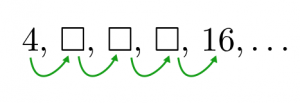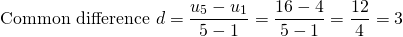# Slope

We can easily see that some line segments are steeper than others. Here’s some slope projects to tune in to slope!

You can read all about slope on mathisfun here or watch this introduction to slope here.

Consider the arithmetic sequenceThe common difference between consecutive terms is 3. The sequence is going up in threes.

Suppose you were given only two terms of the sequence as follows:We can still figure out that the common difference is 3. We see that the sequence value increased from 4 to 16, that is by 12, over four steps:and.In arithmetic sequences, we use the letterto represent common difference.

In linear equations, we call the increase infor every one unit increase on‘slope’; we use the letterand we calculate it in the same way.

# Calculating Slope using Two Points

The formula for finding the slope of a straight line between (or through) two points is:One point has coordinates, the other has coordinates. It doesn’t matter which one is which, as long as whichever point is first on the top line is also first on the bottom line.

Slope is where arithmetic meets geometry. We know that when we count in 8’s, we get higher faster than if we count in 3’s.

A line that goes up 8 units for every one horizontal unit gets higher faster than the line that goes up 3 units for every one unit across.

Slope is a number that represents how quickly a line segment goes up a grid. Slope ‘8’ means that it goes up in 8’s. The grid may or may not be drawn. What matters are the coordinates.

# Move the line segment to create a certain slope

When a line or line segment is drawn on a 1:1 grid, we may simply count boxes to calculate slope:Use the applet to create line segments of the following slopes:

1. Slope AB = 5
2. SlopeAB = -3
3. Slope AB = 0
4. Slope AB = 1
5. Slope AB = -1
6. Infinitely steep
7. Slope AB =8. Slope AB =